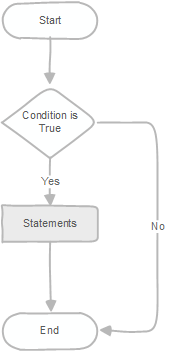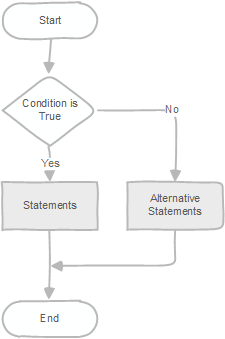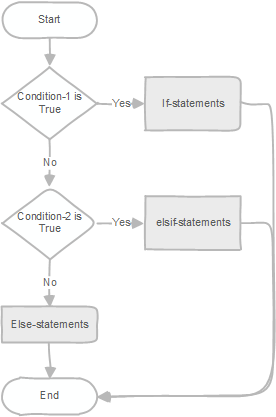# PostgreSQL Tutorial: PL/pgSQL IF Statement

August 4, 2023

Summary: in this tutorial, you will learn how to use the PL/pgSQL `if` statements to execute a command based on a specific condition.

The `if` statement determines which statements to execute based on the result of a boolean expression.

PL/pgSQL provides you with three forms of the `if` statements.

• `if then`
• `if then else`
• `if then elsif`

## 1) PL/pgSQL if-then statement

The following illustrates the simplest form of the `if` statement:

``````if condition then
statements;
end if;
``````

The `if` statement executes `statements` if a `condition` is true. If the `condition` evaluates to `false`, the control is passed to the next statement after the `END if` part.

The `condition` is a boolean expression that evaluates to true or false.

The `statements` can be one or more statements that will be executed if the `condition` is true. It can be any valid statement, even another `if` statement.

When an `if` statement is placed inside another `if` statement, it is called a nested-if statement.

The following flowchart illustrates the simple `if` statement.See the following example:

``````do \$\$
declare
selected_film film%rowtype;
input_film_id film.film_id%type := 0;
begin
select * from film
into selected_film
where film_id = input_film_id;

raise notice 'The film % could not be found',
input_film_id;
end if;
end \$\$;
``````

In this example, we selected a film by a specific film id (`0`).

The `found` is a global variable that is available in PL/pgSQL procedure language. If the `select into` statement sets the found variable if a row is assigned or `false` if no row is returned.

We used the `if` statement to check if the film with id (0) exists and raise a notice if it does not.

``````ERROR:  The film 0 could not be found
``````

If you change the value of the `input_film_id` variable to some value that exists in the film table like 100, you will not see any message.

## 2) PL/pgSQL if-then-else statement

The following illustrates the syntax of the `if-then-else` statement:

``````if condition then
statements;
else
alternative-statements;
end if;
``````

The `if then else` statement executes the statements in the `if` branch if the `condition` evaluates to true; otherwise, it executes the statements in the `else` branch.

The following flowchart illustrates the `if else` statement.See the following example:

``````do \$\$
declare
selected_film film%rowtype;
input_film_id film.film_id%type := 100;
begin
select * from film
into selected_film
where film_id = input_film_id;

raise notice 'The film % could not be found',
input_film_id;
else
raise notice 'The film title is %', selected_film.title;
end if;
end \$\$;
``````

In this example, the film id 100 exists in the film table so that the `FOUND` variable was set to true. Therefore, the statement in the `else` branch executed.

Here is the output:

``````NOTICE:  The film title is Brooklyn Desert
``````

## 3) PL/pgSQL if-then-elsif Statement

The following illustrates the syntax of the `if then elsif` statement:

``````if condition_1 then
statement_1;
elsif condition_2 then
statement_2
...
elsif condition_n then
statement_n;
else
else-statement;
end if;
``````

The `if` and `if then else` statements evaluate one condition. However, the `if then elsif` statement evaluates multiple conditions.

If a `condition` is true, the corresponding statement in that branch is executed.

For example, if the `condition_1` is `true` then the `if then elsif` executes the `statement_1` and stops evaluating the other conditions.

If all conditions evaluate to `false`, the `if then elsif` executes the statements in the `else` branch.

The following flowchart illustrates the `if then elsif` statement:Let’s look at the following example:

``````do \$\$
declare
v_film film%rowtype;
len_description varchar(100);
begin
select * from film
into v_film
where film_id = 100;

else
if v_film.length > 0 and v_film.length <= 50 then
len_description := 'Short';
elsif v_film.length > 50 and v_film.length < 120 then
len_description := 'Medium';
elsif v_film.length > 120 then
len_description := 'Long';
else
len_description := 'N/A';
end if;

raise notice 'The % film is %.',
v_film.title, len_description;
end if;
end \$\$;
``````

How it works:

• First, select the film with id 100. If the film does not exist, raise a notice that the film is not found.
• Second, use the `if then elsif` statement to assign the film a description based on the length of the film.

## Summary

• Use the `if then` to execute statements when a condition is `true`.
• Use the `if then else` to execute statements when a condition is `true` and execute other statements when the condition is `false`.
• Use the `if then elsif` to evaluate multiple conditions and execute statements when the corresponding condition is `true`.